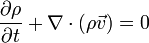User:Tohline/Math/EQ Continuity02$~\frac{\partial\rho}{\partial t} + \nabla \cdot (\rho \vec{v}) = 0$